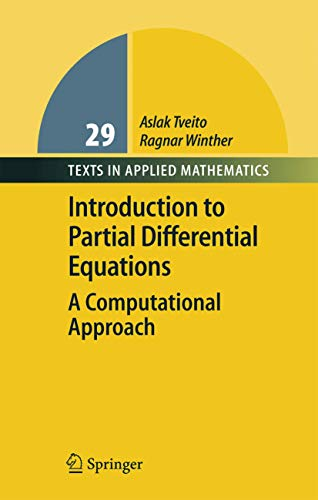Free Shipping in Australia# Introduction to Partial Differential Equations by Aslak Tveito

Used Price
\$72.99
inc. GST
Free Shipping in Australia

This is the softcover reprint of a popular book teaching the basic analytical and computational methods of partial differential equations. It includes coverage of standard topics such as separation of variables, Fourier analysis, and energy estimates.

Only 1 left

## Introduction to Partial Differential Equations Summary

### Introduction to Partial Differential Equations: A Computational Approach by Aslak Tveito

This book teaches basic methods of partial differential equations and introduces related important ideas associated with the analysis of numerical methods for those partial differential equations. Coverage details such topics as separation of variables, Fourier analysis, maximum principles, and energy estimates. The book introduces numerical methods in parallel to the classical theory and also includes many engaging exercises.

### Why buy from World of BooksOur excellent value books literally don't cost the earthFree delivery in AustraliaEvery used book bought is one saved from landfill

## Introduction to Partial Differential Equations Reviews

From the reviews:

"Presents an introductory course to study partial differential equations especially from the computational point of view. ... At the end of each chapter the reader can find a very good selection of fine exercises or projects ... . The language of the book is simple and easy to read which will be highly appreciated by its readers. The book can be very useful for undergraduate and graduate students in mathematics and engineering." (Angela Handlovicova, Zentralblatt MATH, 1063, 2005)

Setting the Scene.- Two-Point Boundary Value Problems.- The Heat Equation.- Finite Difference Schemes for the Heat Equation.- The Wave Equation.- Maximum Principles.- Poisson's Equation in Two Space Dimensions.- Orthogonality and General Fourier Series.- Convergence of Fourier Series.- The Heat Equation Revisited.- Reaction-Diffusion Equations.- Applications of the Fourier Transform.

GOR010332600
Introduction to Partial Differential Equations: A Computational Approach by Aslak Tveito
Aslak Tveito
Texts in Applied Mathematics
Used - Very Good
Hardback
Springer-Verlag Berlin and Heidelberg GmbH & Co. KG
2004-10-04
394
354022551X
9783540225515
N/A
Book picture is for illustrative purposes only, actual binding, cover or edition may vary.
This is a used book - there is no escaping the fact it has been read by someone else and it will show signs of wear and previous use. Overall we expect it to be in very good condition, but if you are not entirely satisfied please get in touch with us.